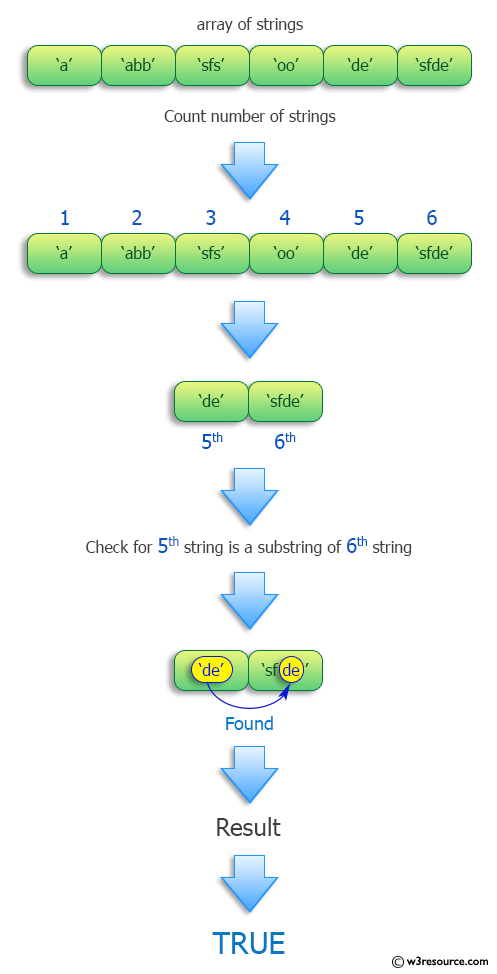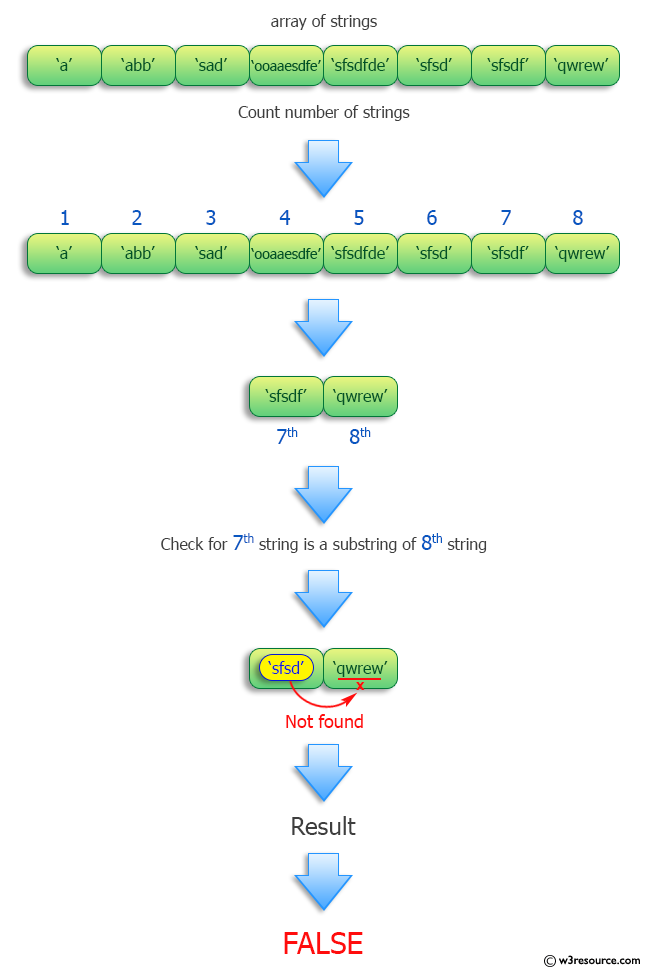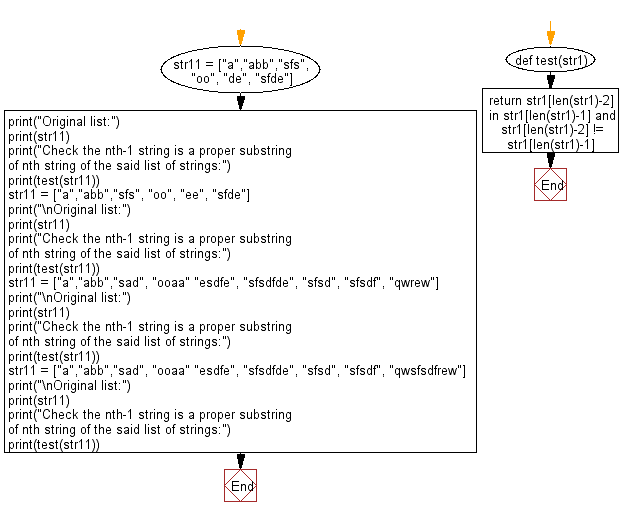﻿ Python: Check the nth-1 string is a proper substring of nth string of a given list of strings - w3resource# Python: Check the nth-1 string is a proper substring of nth string of a given list of strings

## Python Programming Puzzles: Exercise-5 with Solution

Write a Python program to check the nth-1 string is a proper substring of nth string in a given list of strings.

```Input:
['a', 'abb', 'sfs', 'oo', 'de', 'sfde']
Output:
True

Input:
['a', 'abb', 'sfs', 'oo', 'ee', 'sfde']
Output:
False

Input:
['a', 'abb', 'sad', 'ooaaesdfe', 'sfsdfde', 'sfsd', 'sfsdf', 'qwrew']
Output:
False

Input:
['a', 'abb', 'sad', 'ooaaesdfe', 'sfsdfde', 'sfsd', 'sfsdf', 'qwsfsdfrew']
Output:
True```

Pictorial Presentation:Sample Solution:

Python Code:

``````#License: https://bit.ly/3oLErEI

def test(str1):
return str1[len(str1)-2] in str1[len(str1)-1] and str1[len(str1)-2] != str1[len(str1)-1]

str11 = ["a","abb","sfs", "oo", "de", "sfde"]
print("Original list:")
print(str11)
print("Check the nth-1 string is a proper substring of nth string of the said list of strings:")
print(test(str11))
str11 = ["a","abb","sfs", "oo", "ee", "sfde"]
print("\nOriginal list:")
print(str11)
print("Check the nth-1 string is a proper substring of nth string of the said list of strings:")
print(test(str11))
str11 = ["a","abb","sad", "ooaa" "esdfe", "sfsdfde", "sfsd", "sfsdf", "qwrew"]
print("\nOriginal list:")
print(str11)
print("Check the nth-1 string is a proper substring of nth string of the said list of strings:")
print(test(str11))
str11 = ["a","abb","sad", "ooaa" "esdfe", "sfsdfde", "sfsd", "sfsdf", "qwsfsdfrew"]
print("\nOriginal list:")
print(str11)
print("Check the nth-1 string is a proper substring of nth string of the said list of strings:")
print(test(str11))
``````

Sample Output:

```Original list:
['a', 'abb', 'sfs', 'oo', 'de', 'sfde']
Check the nth-1 string is a proper substring of nth string of the said list of strings:
True

Original list:
['a', 'abb', 'sfs', 'oo', 'ee', 'sfde']
Check the nth-1 string is a proper substring of nth string of the said list of strings:
False

Original list:
['a', 'abb', 'sad', 'ooaaesdfe', 'sfsdfde', 'sfsd', 'sfsdf', 'qwrew']
Check the nth-1 string is a proper substring of nth string of the said list of strings:
False

Original list:
['a', 'abb', 'sad', 'ooaaesdfe', 'sfsdfde', 'sfsd', 'sfsdf', 'qwsfsdfrew']
Check the nth-1 string is a proper substring of nth string of the said list of strings:
True
```

Flowchart:## Visualize Python code execution:

The following tool visualize what the computer is doing step-by-step as it executes the said program:

Python Code Editor :

Have another way to solve this solution? Contribute your code (and comments) through Disqus.

What is the difficulty level of this exercise?

Test your Programming skills with w3resource's quiz.

﻿

## Python: Tips of the Day

Clamps num within the inclusive range specified by the boundary values x and y:

Example:

```def tips_clamp_num(num,x,y):
return max(min(num, max(x, y)), min(x, y))
print(tips_clamp_num(2, 4, 6))
print(tips_clamp_num(1, -1, -6))
```

Output:

```4
-1
```